Design Optimization of a Welded Beam

This example shows how to examine the tradeoff between the strength and cost of a beam. Several publications use this example as a test problem for various multiobjective algorithms, including Deb et al.  and Ray and Liew .

For a video overview of this example, see Pareto Sets for Multiobjective Optimization.

Problem Description

The following sketch is adapted from Ray and Liew .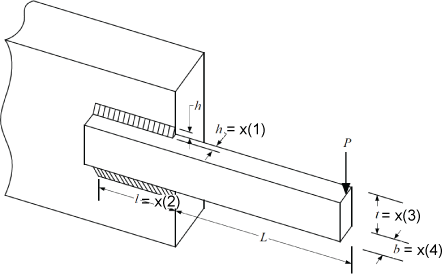This sketch represents a beam welded onto a substrate. The beam supports a load P at a distance L from the substrate. The beam is welded onto the substrate with upper and lower welds, each of length l and thickness h. The beam has a rectangular cross-section, width b, and height t. The material of the beam is steel.

The two objectives are the fabrication cost of the beam and the deflection of the end of the beam under the applied load P. The load P is fixed at 6,000 lbs, and the distance L is fixed at 14 in.

The design variables are:

• x(1) = h, the thickness of the welds

• x(2) = l, the length of the welds

• x(3) = t, the height of the beam

• x(4) = b, the width of the beam

The fabrication cost of the beam is proportional to the amount of material in the beam, $\left(l+L\right)tb$, plus the amount of material in the welds, $l{h}^{2}$. Using the proportionality constants from the cited papers, the first objective is

${F}_{1}\left(x\right)=1.10471{x}_{1}^{2}{x}_{2}+0.04811{x}_{3}{x}_{4}\left(14+{x}_{2}\right).$

The deflection of the beam is proportional to P and inversely proportional to $b{t}^{3}$. Again, using the proportionality constants from the cited papers, the second objective is

${F}_{2}\left(x\right)=\frac{P}{{x}_{4}{x}_{3}^{3}}C$, where $C=\frac{4\left(14{\right)}^{3}}{30×1{0}^{6}}\approx 3.6587×1{0}^{-4}$ and $\mathit{P}=6,000$.

The problem has several constraints.

• The weld thickness cannot exceed the beam width. In symbols, x(1) <= x(4). In toolbox syntax:

Aineq = [1,0,0,-1];
bineq = 0;
• The shear stress $\tau \left(x\right)$ on the welds cannot exceed 13,600 psi. To calculate the shear stress, first calculate preliminary expressions:

${\tau }_{1}=\frac{1}{\sqrt{2}{x}_{1}{x}_{2}}$

$R=\sqrt{{x}_{2}^{2}+\left({x}_{1}+{x}_{3}{\right)}^{2}}$

${\tau }_{2}=\frac{\left(L+{x}_{2}/2\right)R}{\sqrt{2}{x}_{1}{x}_{3}\left({x}_{2}^{2}/3+\left({x}_{1}+{x}_{3}{\right)}^{2}}$

$\tau \left(x\right)=P\sqrt{{\tau }_{1}^{2}+{\tau }_{2}^{2}+\frac{2{\tau }_{1}{\tau }_{2}{x}_{2}}{R}}.$

In summary, the shear stress on the welds has the constraint $\tau \left(x\right)$ <= 13600.

• The normal stress $\sigma \left(x\right)$ on the welds cannot exceed 30,000 psi. The normal stress is $P\frac{6L}{{x}_{4}{x}_{3}^{2}}\le 30×1{0}^{3}$.

• The buckling load capacity in the vertical direction must exceed the applied load of 6,000 lbs. Using the values of Young's modulus $E=30×1{0}^{6}$ psi and $G=12×1{0}^{6}$ psi, the buckling load constraint is $\frac{4.013E{x}_{3}{x}_{4}^{3}}{6{L}^{2}}\left(1-\frac{{x}_{3}}{2L}\sqrt{\frac{E}{4G}}\right)\ge 6000$. Numerically, this becomes the inequality $64,746.022\left(1-0.0282346\phantom{\rule{0.16666666666666666em}{0ex}}{x}_{3}\right){x}_{3}{x}_{4}^{3}\ge 6000$.

• The bounds on the variables are 0.125 <=x(1) <= 5, 0.1 <= x(2) <= 10, 0.1 <= x(3) <= 10, and 0.125 <= x(4) <= 5. In toolbox syntax:

lb = [0.125,0.1,0.1,0.125];
ub = [5,10,10,5];

The objective functions appear at the end of this example in the function objval(x). The nonlinear constraints appear at the end of this example in the function nonlcon(x).

Multiobjective Problem Formulation and paretosearch Solution

You can optimize this problem in several ways:

• Set a maximum deflection, and find a single-objective minimal fabrication cost over designs that satisfy the maximum deflection constraint.

• Set a maximum fabrication cost, and find a single-objective minimal deflection over designs that satisfy the fabrication cost constraint.

• Solve a multiobjective problem, visualizing the tradeoff between the two objectives.

To use the multiobjective approach, which gives more information about the problem, set the objective function and nonlinear constraint function.

fun = @objval;
nlcon = @nonlcon;

Solve the problem using paretosearch with the 'psplotparetof' plot function. To reduce the amount of diagnostic display information, set the Display option to 'off'.

opts_ps = optimoptions('paretosearch','Display','off','PlotFcn','psplotparetof');
rng default % For reproducibility
[x_ps1,fval_ps1,~,psoutput1] = paretosearch(fun,4,Aineq,bineq,[],[],lb,ub,nlcon,opts_ps);disp("Total Function Count: " + psoutput1.funccount);
Total Function Count: 1870

For a smoother Pareto front, try using more points.

npts = 160; % The default is 60
opts_ps.ParetoSetSize = npts;
[x_ps2,fval_ps2,~,psoutput2] = paretosearch(fun,4,Aineq,bineq,[],[],lb,ub,nlcon,opts_ps);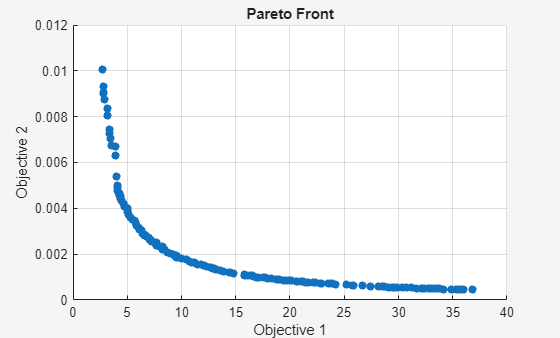disp("Total Function Count: " + psoutput2.funccount);
Total Function Count: 6254

This solution looks like a smoother curve, but it has a smaller extent of Objective 2. The solver takes over three times as many function evaluations when using 160 Pareto points instead of 60.

gamultiobj Solution

To see if the solver makes a difference, try the gamultiobj solver on the problem. Set equivalent options as in the previous solution. Because the gamultiobj solver keeps fewer than half of its solutions on the best Pareto front, use two times as many points as before.

opts_ga = optimoptions('gamultiobj','Display','off','PlotFcn','gaplotpareto','PopulationSize',2*npts);
[x_ga1,fval_ga1,~,gaoutput1] = gamultiobj(fun,4,Aineq,bineq,[],[],lb,ub,nlcon,opts_ga);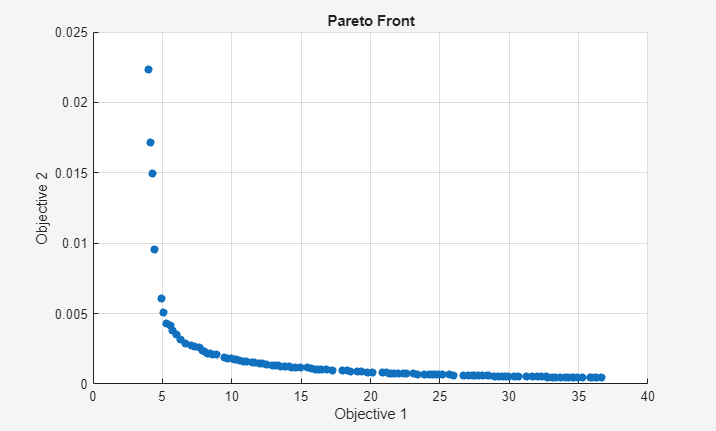disp("Total Function Count: " + gaoutput1.funccount);
Total Function Count: 38401

gamultiobj takes tens of thousands of function evaluations, whereas paretosearch takes only thousands.

Compare Solutions

The gamultiobj solution seems to differ from the paretosearch solution, although it is difficult to tell because the plotted scales differ. Plot the two solutions on the same plot, using the same scale.

fps2 = sortrows(fval_ps2,1,'ascend');
figure
hold on
plot(fps2(:,1),fps2(:,2),'r-')
fga = sortrows(fval_ga1,1,'ascend');
plot(fga(:,1),fga(:,2),'b--')
xlim([0,40])
ylim([0,1e-2])
legend('paretosearch','gamultiobj')
xlabel 'Cost'
ylabel 'Deflection'
hold off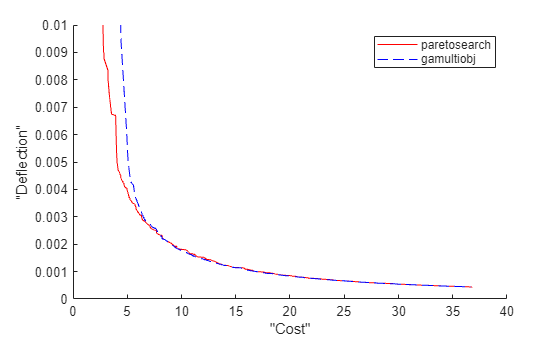The gamultiobj solution is better in the rightmost portion of the plot, whereas the paretosearch solution is better in the leftmost portion. paretosearch uses many fewer function evaluations to obtain its solution.

Typically, when the problem has no nonlinear constraints, paretosearch is at least as accurate as gamultiobj. However, the resulting Pareto sets can have somewhat different ranges. In this case, the presence of a nonlinear constraint causes the paretosearch solution to be less accurate over part of the range.

One of the main advantages of paretosearch is that it usually takes many fewer function evaluations.

Start from Single-Objective Solutions

To help the solvers find better solutions, start them from points that are the solutions to minimizing the individual objective functions. The pickindex function returns a single objective from the objval function. Use fmincon to find single-objective optima. Then use those solutions as initial points for a multiobjective search.

x0 = zeros(2,4);
x0f = (lb + ub)/2;
opts_fmc = optimoptions('fmincon','Display','off','MaxFunctionEvaluations',1e4);
x0(1,:) = fmincon(@(x)pickindex(x,1),x0f,Aineq,bineq,[],[],lb,ub,@nonlcon,opts_fmc);
x0(2,:) = fmincon(@(x)pickindex(x,2),x0f,Aineq,bineq,[],[],lb,ub,@nonlcon,opts_fmc);

Examine the single-objective optima.

objval(x0(1,:))
ans = 1×2

2.3810    0.0158

objval(x0(2,:))
ans = 1×2

76.7188    0.0004

The minimum cost is 2.381, which gives a deflection of 0.158. The minimum deflection is 0.0004, which has a cost of 76.7253. The plotted curves are quite steep near the ends of their ranges, meaning you get much less deflection if you take a cost a bit above its minimum, or much less cost if you take a deflection a bit above its minimum.

Start paretosearch from the single-objective solutions. Because you will plot the solutions later on the same plot, remove the paretosearch plot function.

opts_ps.InitialPoints = x0;
opts_ps.PlotFcn = [];
[x_psx0,fval_ps1x0,~,psoutput1x0] = paretosearch(fun,4,Aineq,bineq,[],[],lb,ub,nlcon,opts_ps);
disp("Total Function Count: " + psoutput1x0.funccount);
Total Function Count: 4839

Start ga from the same initial points, and remove its plot function.

opts_ga.InitialPopulationMatrix = x0;
opts_ga.PlotFcn = [];
[~,fval_ga,~,gaoutput] = gamultiobj(fun,4,Aineq,bineq,[],[],lb,ub,nlcon,opts_ga);
disp("Total Function Count: " + gaoutput.funccount);
Total Function Count: 37441

Plot the solutions on the same axes.

fps = sortrows(fval_ps1x0,1,'ascend');
figure
hold on
plot(fps(:,1),fps(:,2),'r-')
fga = sortrows(fval_ga,1,'ascend');
plot(fga(:,1),fga(:,2),'b--')
xlim([0,40])
ylim([0,1e-2])
legend('paretosearch','gamultiobj')
xlabel 'Cost'
ylabel 'Deflection'
hold off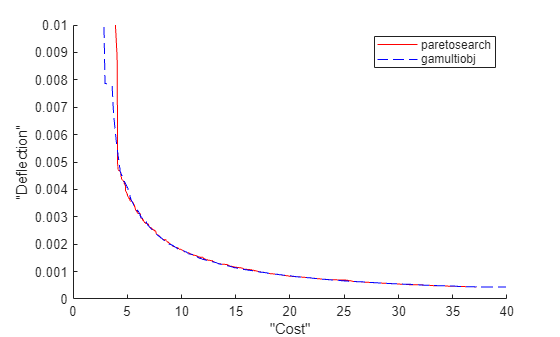By starting from the single-objective solutions, the gamultiobj solution is slightly better than the paretosearch solution throughout the plotted range. However, gamultiobj takes almost ten times as many function evaluations to reach its solution.

Hybrid Function

gamultiobj can call the hybrid function fgoalattain automatically to attempt to reach a more accurate solution. See whether the hybrid function improves the solution.

opts_ga.HybridFcn = 'fgoalattain';
[xgah,fval_gah,~,gaoutputh] = gamultiobj(fun,4,Aineq,bineq,[],[],lb,ub,nlcon,opts_ga);
disp("Total Function Count: " + gaoutputh.funccount);
Total Function Count: 57478
fgah = sortrows(fval_gah,1,'ascend');
figure
hold on
plot(fps(:,1),fps(:,2),'r-')
plot(fga(:,1),fga(:,2),'b--')
plot(fgah(:,1),fgah(:,2),'g-')
xlim([0,40])
ylim([0,1e-2])
legend('paretosearch','gamultiobj','gamultiobj/fgoalattain')
xlabel 'Cost'
ylabel 'Deflection'
hold offThe hybrid function provides a slight improvement on the gamultiobj solution, mainly in the leftmost part of the plot.

Run fgoalattain Manually from paretosearch Solution Points

Although paretosearch has no built-in hybrid function, you can improve the paretosearch solution by running fgoalattain from the paretosearch solution points. Create a goal and weights for fgoalattain by using the same setup for fgoalattain as described in gamultiobj Hybrid Function.

Fmax = max(fval_ps1x0);
nobj = numel(Fmax);
Fmin = min(fval_ps1x0);
w = sum((Fmax - fval_ps1x0)./(1 + Fmax - Fmin),2);
p = w.*((Fmax - fval_ps1x0)./(1 + Fmax - Fmin));
xnew = zeros(size(x_psx0));
nsol = size(xnew,1);
fvalnew = zeros(nsol,nobj);
opts_fg = optimoptions('fgoalattain','Display','off');
nfv = 0;
for ii = 1:nsol
[xnew(ii,:),fvalnew(ii,:),~,~,output] = fgoalattain(fun,x_psx0(ii,:),fval_ps1x0(ii,:),p(ii,:),...
Aineq,bineq,[],[],lb,ub,nlcon,opts_fg);
nfv = nfv + output.funcCount;
end
disp("fgoalattain Function Count: " + nfv)
fgoalattain Function Count: 14049
fnew = sortrows(fvalnew,1,'ascend');
figure
hold on
plot(fps(:,1),fps(:,2),'r-')
plot(fga(:,1),fga(:,2),'b--')
plot(fgah(:,1),fgah(:,2),'g-')
plot(fnew(:,1),fnew(:,2),'k.-')
xlim([0,40])
ylim([0,1e-2])
legend('paretosearch','gamultiobj','gamultiobj/fgoalattain','paretosearch/fgoalattain')
xlabel 'Cost'
ylabel 'Deflection'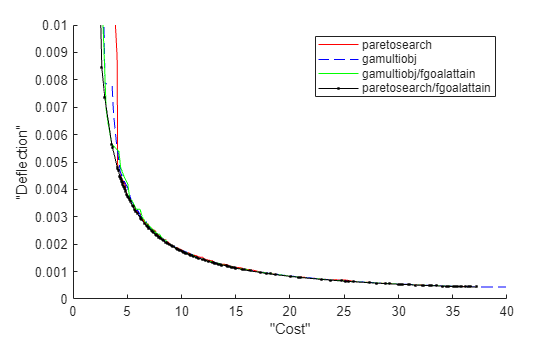The combination of paretosearch and fgoalattain creates the most accurate Pareto front. Zoom in to see.

xlim([3.64 13.69])
ylim([0.00121 0.00442])
hold off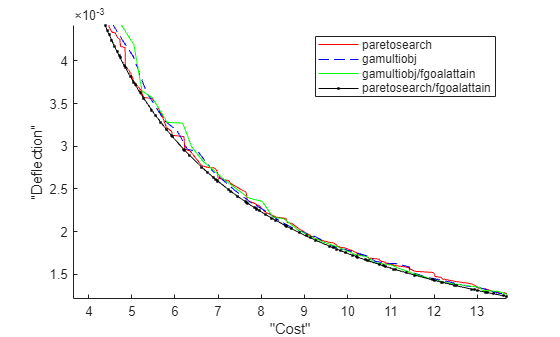Even with the extra fgoalattain computations, the total function count for the combination is less than half of the function count for the gamultiobj solution alone.

fprintf("Total function count for gamultiobj alone is %d.\n" + ...
"For paretosearch and fgoalattain together it is %d.\n",...
gaoutput.funccount,nfv + psoutput1x0.funccount)
Total function count for gamultiobj alone is 37441.
For paretosearch and fgoalattain together it is 18888.

Find Good Parameters from Plot

The plotted points show the best values in function space. To determine which parameters achieve these function values, find the size of the beam and size of the weld in order to get a particular cost/deflection point. For example, the plot of paretosearch followed by fgoalattain shows points with a cost of about 6 and a deflection of about 3.5e–3. Determine the sizes of the beam and weld that achieve these points.

whichgood = find(fvalnew(:,1) <= 6 & fvalnew(:,2) <= 3.5e-3);
goodpoints = table(xnew(whichgood,:),fvalnew(whichgood,:),'VariableNames',{'Parameters' 'Objectives'})
goodpoints=4×2 table
Parameters                       Objectives
________________________________________    ___________________

0.63457     1.5187         10    0.67262    5.6974    0.0032637
0.61635     1.5708         10    0.63165     5.391    0.0034753
0.63228     1.5251         10     0.6674    5.6584    0.0032892
0.65077     1.4751         10    0.70999     5.976    0.0030919

Four sets of parameters achieve a cost of less than 6 and a deflection of less than 3.5e–3:

• Weld thickness slightly over 0.6

• Beam height 10 (the upper bound)

• Beam width between 0.63 and 0.71

Objective and Nonlinear Constraints

function [Cineq,Ceq] = nonlcon(x)
sigma = 5.04e5 ./ (x(:,3).^2 .* x(:,4));
P_c = 64746.022*(1 - 0.028236*x(:,3)).*x(:,3).*x(:,4).^3;
tp = 6e3./sqrt(2)./(x(:,1).*x(:,2));
tpp = 6e3./sqrt(2) .* (14+0.5*x(:,2)).*sqrt(0.25*(x(:,2).^2 + (x(:,1) + x(:,3)).^2)) ./ (x(:,1).*x(:,2).*(x(:,2).^2 / 12 + 0.25*(x(:,1) + x(:,3)).^2));
tau = sqrt(tp.^2 + tpp.^2 + (x(:,2).*tp.*tpp)./sqrt(0.25*(x(:,2).^2 + (x(:,1) + x(:,3)).^2)));
Cineq = [tau - 13600,sigma - 3e4,6e3 - P_c];
Ceq = [];
end

function F = objval(x)
f1 = 1.10471*x(:,1).^2.*x(:,2) + 0.04811*x(:,3).*x(:,4).*(14.0+x(:,2));
f2 = 2.1952./(x(:,3).^3 .* x(:,4));

F = [f1,f2];
end

function z = pickindex(x,k)
z = objval(x); % evaluate both objectives
z = z(k); % return objective k
end

References

 Deb, Kalyanmoy, J. Sundar, Udaya Bhaskara Rao N, and Shamik Chaudhuri. Reference Point Based Multi-Objective Optimization Using Evolutionary Algorithms. International Journal of Computational Intelligence Research, Vol. 2, No. 3, 2006, pp. 273–286. Available at https://www.softcomputing.net/ijcir/vol2-issu3-paper4.pdf

 Ray, T., and K. M. Liew. A Swarm Metaphor for Multiobjective Design Optimization. Engineering Optimization 34, 2002, pp.141–153.

Global Optimization Toolbox DocumentationGet trial now